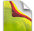### Feel The Power# PHP Arrays

An array is a data structure that stores one or more similar type of values in a single value. For example if you want to store 100 numbers then instead of defining 100 variables its easy to define an array of 100 length.

There are three different kind of arrays and each array value is accessed using an ID c which is called array index.

• Numeric array − An array with a numeric index. Values are stored and accessed in linear fashion.

• Associative array − An array with strings as index. This stores element values in association with key values rather than in a strict linear index order.

• Multidimensional array − An array containing one or more arrays and values are accessed using multiple indices

NOTE − Built-in array functions is given in function reference PHP Array Functions

## Numeric Array

These arrays can store numbers, strings and any object but their index will be represented by numbers. By default array index starts from zero.

### Example

Following is the example showing how to create and access numeric arrays.

Here we have used array() function to create array. This function is explained in function reference.

``````<html>
<body>

<?php
/* First method to create array. */
\$numbers = array( 1, 2, 3, 4, 5);

foreach( \$numbers as \$value ) {
echo "Value is \$value <br />";
}

/* Second method to create array. */
\$numbers = "one";
\$numbers = "two";
\$numbers = "three";
\$numbers = "four";
\$numbers = "five";

foreach( \$numbers as \$value ) {
echo "Value is \$value <br />";
}
?>

</body>
</html>``````

## Associative Arrays

The associative arrays are very similar to numeric arrays in term of functionality but they are different in terms of their index. Associative array will have their index as string so that you can establish a strong association between key and values.

To store the salaries of employees in an array, a numerically indexed array would not be the best choice. Instead, we could use the employees names as the keys in our associative array, and the value would be their respective salary.

NOTE − Don't keep associative array inside double quote while printing otherwise it would not return any value.

### Example

``````<html>
<body>

<?php
/* First method to associate create array. */

echo "Salary of zara is ".  \$salaries['zara']. "<br />";

/* Second method to create array. */
\$salaries['zara'] = "low";

echo "Salary of zara is ".  \$salaries['zara']. "<br />";
?>

</body>
</html>``````

## Multidimensional Arrays

A multi-dimensional array each element in the main array can also be an array. And each element in the sub-array can be an array, and so on. Values in the multi-dimensional array are accessed using multiple index.

### Example

In this example we create a two dimensional array to store marks of three students in three subjects −

This example is an associative array, you can create numeric array in the same fashion.

``````<html>
<body>

<?php
\$marks = array(
"physics" => 35,
"maths" => 30,
"chemistry" => 39
),

"physics" => 30,
"maths" => 32,
"chemistry" => 29
),

"zara" => array (
"physics" => 31,
"maths" => 22,
"chemistry" => 39
)
);

/* Accessing multi-dimensional array values */
echo "Marks for mohammad in physics : " ;

echo "Marks for qadir in maths : ";

echo "Marks for zara in chemistry : " ;
echo \$marks['zara']['chemistry'] . "<br />";
?>

</body>
</html>``````

#

#

#

### PHP Arrays Examples

TOP TUTORIALS
WHAT IS NEW IN KERIXA?
RECENT TUTORIALS
CATEGORIES